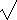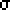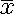# Hypothesis Testing

### Hypothesis Testing

Once descriptive statistics, combinatorics, and distributions are well understood, we can move on to the vast area of inferential statistics. The basic concept is one called hypothesis testing or sometimes the test of a statistical hypothesis. Here we have two conflicting theories about the value of a population parameter. It is very important that the hypotheses be conflicting (contradictory), if one is true, the other must be false and vice versa. Another way to say this is that they are mutually exclusive and exhaustive, that is, no overlap and no other values are possible. Simple hypotheses only test against one value of the population parameter (p=½, for instance), whereas composite hypotheses test a range of values (p > ½).

Our two hypotheses have special names: the null hypothesis represented by H0 and the alternative hypothesis by Ha. Historically, the null (invalid, void, amounting to nothing) hypothesis was what the researcher hoped to reject. These days it is common practice not to associate any special meaning to which hypothesis is which. (But this common practice may not yet have extended into behavioral science. The research hypothesis becomes the alternate hypothesis and the null hypothesis or "straw man" to be knocked down is so determined.) Although simple hypotheses would be easiest to test, it is much more common to have one of each type or perhaps for both to be composite. If the values specified by Ha are all on one side of the value specified by H0, then we have a one-sided test (one-tailed), whereas if the Ha values lie on both sides of H0, then we have a two-sided test (two-tailed). A one-tailed test is sometimes called a directional test and a two-tailed test is sometimes called a nondirectional test.

The outcome of our test regarding the population parameter will be that we either reject the null hypothesis or fail to reject the null hypothesis. It is considered poor form to "accept" the null hypothesis, although if we fail to reject it, that is in fact essentially what we are doing. When we reject the null hypothesis we have only shown that it is highly unlikely to be true---we have not proven it in the mathematical sense. The research hypothesis is supported by rejecting the null hypothesis. The null hypothesis locates the sampling distribution, since it is (usually) the simple hypothesis, testing against one specific value of the population parameter. Establishing the null and alternative hypotheses is sometimes considered the first step in hypothesis testing.

### Type I and Type II Errors

Two types of errors can occur and there are three naming schemes for them. These errors cannot both occur at once. Perhaps a table will make it clearer.

Reject\Truth H0 True no error False positive, Type II, beta=P(Reject Ha|Ha true) False negative, Type I, alpha=P(Reject H0|H0 true) no error

The term false positive for type II errors comes from perhaps a blood test where the test results came back positive, but it is not the case (false) that the person has whatever was being tested for. The term false negative for type I errors then would mean that the person does indeed have whatever was being tested for, but the test didn't find it. When testing for pregnancy, AIDS, or other medical conditions, both types of errors can be a very serious matter. Formally, alpha=P(Accept Ha|H0 true), meaning the probability that we "accepted" Ha when in fact H0 was true. Alpha is the term used to express the level of significance we will accept. For 95% confidence, alpha=0.05. For 99% confidence, alpha=0.01. These two alpha values are the ones most frequently used. If our P-value, the high unlikeliness of the H0, is less than alpha, we can reject the null hypothesis. Alpha and beta usually cannot both be minimized---there is a trade-off between the two. Ideally, of course, we would minimize both. Historically, a fixed level of significance was selected (alpha=0.05 for the social sciences and alpha=0.01 or alpha=0.001 for the natural sciences, for instance). This was due to the fact that the null hypothesis was considered the "current theory" and the size of Type I errors was much more important than that of Type II errors. Now both are usually considered together when determining an adequately sized sample. Instead of testing against a fixed level of alpha, now the P-value is often reported. Obviously, the smaller the P-value, the stronger the evidence (higher significance, smaller alpha) provided by the data is against H0.

Example: On July 14, 2005 we took 10 samples of 20 pennies set on edge and the table banged. The resultant mean of heads was 14.5 with a standard deviation of 2.12. Since this is a small sample, and the population variance is unknown, after we calculate a t value and obtain t=6.71=(14.5-10)/(2.12/(10)), we apply the t-test and find a P-value of either 8.73×10-5 or 4.36×10-5 depending on whether we do a one-tailed or two-tailed test. In either case our results are statistically significant at the 0.0001 level.

 The P-value of a test is the probability that the test statistic would take a value as extreme or more extreme than that actually observed, assuming H0 is true.

### Power of a Test

The power of a test against the associated correct value is 1-beta. It is the probability that a Type II error is not committed. There is a different value of beta for each possible correct value of the population parameter. It also depends on sample size (n), thus increasing the sample size increases the power. Power is thus important in planning and interpretting tests of significance. It is easy to misspeak power (1-beta) and P-value (alpha). Power will be examined in greater detail in lesson 11 (Hinkle chapter 13).

Setting the level of significance will correspond to the probability that we are willing to be wrong in our conclusion if a type I error was committed. That probability will correspond to certain area(s) under the curve of a probability distribution. Those areas, known as the region of rejection is bounded by a critical value or critical values which are often computed. Alternatively, one might compare the test statistic with the corresponding point(s) on the probability curve. These are equivalent ways of viewing the problem, just different units of measure are being used. In a one-tailed test there is one area bounded by one critical value and in a two-tailed test there are two areas bounded by two critical values. Which tail (left or right) under consideration for a one-tailed test depends on the direction of the hypothesis.

Establishing the significance level and the corresponding critical value(s) is sometimes considered the second step in hypothesis testing. Presumeably we have determined how the statistic we wish to test is distributed. This sampling distribution is the underlying distribution of the statistic and determines which statistical test will be performed.

### Computing a Test Statistic

Once the hypotheses have been stated, and the criterion for rejecting the null hypothesis establish, we compute the test statistic. The test statistic for testing a null hypothesis regarding the population mean is a z-score, if the population variance is known (yeah right!). We used a t-score above, which is computing similarly, due to the small size of our sample and the fact that we do not know the population variance. We will have to examine other such test statistics and their underlying distributions. However, the same basic procedure always applies. This is considered by some step 3 in hypothesis testing.

### Making a decision about H0

The last step is whether we reject or fail to reject the null hypothesis. Although it is common to state that we have a small chance that the observed test statistic will occur by chance if the null hypothesis true, it is technically more correct to realize that the statement should refer to a test statistic this extreme or more extreme since the area under any point on the probability curve is zero. It can also be said that the difference between the observed and expected test statistic is too great to be attributed to chance sampling fluctuations. That is 19 out of 20 times it is too great---there is that 1 in 20 chance that our random sample betrayed us (given an alpha=0.05). Again, should we fail to reject the null hypothesis we have to be careful to make the correct statement, such as: the probability that a test statistic of blah would appear by chance, if the population parameter were blah, is greater than 0.05. Stated this way the level of significance used is clear and we have not committed another common error (that with 95% probability, H0 is true).

### Student t Distribution

It is often the case that one wants to calculate the size of sample needed to obtain a certain level of confidence in survey results. Unfortunately, this calculation requires prior knowledge of the population standard deviation (). Realistically,is unknown. Often a preliminary sample will be conducted so that a reasonable estimate of this critical population parameter can be made. If such a preliminary sample is not made, but confidence intervals for the population mean are to be constructing using an unknown, then the distribution known as the Student t distribution can be used.

Testing a hypothesis at the alpha=0.05 level or establishing a 95% confidence interval are again essentially the same thing. In both cases the critical values and the region of rejection are the same. However, we will more formally develop the confidence intervals in lesson 9 (Hinkle chapter 9).

First, a little history about this distribution's curious name. William Gosset (1876-1937) was a Guinness Brewery chemist who needed a distribution that could be used with small samples. Since the Irish brewery did not allow publication of research results, he published in 1908 under the pseudonym of Student. We know that large samples approach a normal distribution. What Gosset showed was that small samples taken from an essentially normal population have a distribution characterized by the sample size. The population does not have to be exactly normal, only unimodal and basically symmetric. This is often characterized as heap-shaped or mound shaped.

Following are the important properties of the Student t distribution.

1. The Student t distribution is different for different sample sizes.
2. The Student t distribution is generally bell-shaped, but with smaller sample sizes shows increased variability (flatter). In other words, the distribution is less peaked than a normal distribution and with thicker tails (platykurtic). As the sample size increases, the distribution approaches a normal distribution. For n > 30, the differences are negligible.
3. The mean is zero (much like the standard normal distribution).
4. The distribution is symmetrical about the mean.
5. The variance is greater than one, but approaches one from above as the sample size increases (2=1 for the standard normal distribution).
6. It takes into account the fact that the population standard deviation is unknown.
7. The population is essentially normal (unimodal and basically symmetric)

To use the Student t distribution which is often referred to just as the t distribution, the first step is to calculate a t-score. This is much like finding the z-score. The formula is:

 t = (- µ) ÷ (s/sqrt(n))

Actually, since the population mean is likely also unknown, the sample mean must be used. The critical t-score can be looked up based on the level of confidence desired and the degrees of freedom. Degrees of freedom is a fairly technical term which permeates all of inferential statistics. It is usually abbreviated df. In this case, it is the very common value n-1.

 In general, the degrees of freedom is the number of values that can vary after certain restrictions have been imposed on all values.

Where does the term degrees of freedom come from? Suppose, for example, that you have a phone bill from Ameritech that says your household owes \$100. Your mother and father state that \$70 of it is theirs and that your younger sibling owes only \$5. How much does that leave you? Here, n=3 (parents, sibling, you), but once you have the total (or mean) and two more pieces of information, the last data element is constrained. The same is true with the degrees of freedom, you can arbitrarily use any n-1 data points, but the last one will be determined for a given mean. Another example is with 10 tests that averaged 55, if you assign nine people random grades, the last test score is not random, but constrained by the overall mean. Thus for 10 tests and a mean, there are nine degrees of freedom.

If the interval calls for a 90% confidence level, then alpha = 0.10 and alpha/2 = 0.05 (for a two-tailed test). Tables of t values typically have a column for degrees of freedom and then columns of t values corresponding with various tail areas. An abbreviated table is given below. For a complete set of values consult a larger table or your TI-83+ graphing calculator. DISTR 5 gives tcdf. tcdf expects three arguments, lower t value, upper t value, and degrees of freedom. Since no inverse t function is given on the calculator, some guessing may be involved. Note how tcdf(9.9,9E99,2) indicates a t value of about 9.9 for a one tailed area of 0.005 with two degrees of freedom. Please locate the corresponding value of 9.925 in the table.

As with other confidence intervals, we use the t-score to obtain the margin of error term which is added and subtracted from the statistic of interest (in this case, the sample mean) to obtain a confidence interval for the parameter of interest (in this case, the population mean). In this case the margin of error is defined (since you don't have population standard deviation you use the sample's) as:

 ME = talpha/2 • (s ÷ sqrt(n))

Your confidence interval should look like:- ME < µ <+ ME.

### Table of t Values

The headings in the table below, such as .005/.01 indicate the left/right tail area (0.005) for a one tail test or the total tail area (left+right=0.01) for a two tailed test. In general, if an entry for the degrees of freedom you desire is not present in the table, use an entry for the next smaller value of the degrees of freedom. This guarantees a conservative estimate.

Degrees of Freedom\1/2 tails .005/.01.01/.02.025/.05.05/.10.10/.20
163.6631.8212.716.3143.078
29.9256.9654.3032.9201.886
35.8414.5413.1822.3531.638
44.6043.7472.7762.1321.533
54.0323.3652.5712.0151.476
103.1692.7642.2281.8121.372
152.9472.6022.1321.7531.341
202.8452.5282.0861.7251.325
252.7872.4852.0601.7081.316
z 2.5762.3261.9601.6451.282

Although the t procedure is fairly robust, that is it does not change very much when the assumptions of the procedure are violated, you should always plot the data to check for skewness and outliers before using it on small samples. Here small can be interpreted as n < 15. If your sample is small and the data is clearly nonnormal or outliers are present, do not use the t. If your sample is not small, but n < 40, and there are outliners or strong skewness, do not use the t. Since the assumption that the samples are random is more important that the normality of the population distribution, the t statistic can be safely used even when the sample indicates the population is clearly skewed, if n > 40.

The two sample t tests will be discussed in lesson 10.

### Practical Importance and Statistical Significance

Not everything which is statistically significant is of practical importance. The choice of alpha (level of significance) is often rather arbitrary. R. A. Fisher used them in agricultural experiments in the early decades of the 1900's and went a long ways toward unifying the field. A medical doctor might easily argue for a smaller alpha than a behavior scientist. Results significant at the 0.10 level might have real meaning that get rejected at the 0.05 level. Thus the field of research and the study's characteristics need careful attention.

Statistical precision can be defined as the reciprocal of the standard error for a given test statistic. Statistical precision is thus influenced directly by sample size, or rather its square root. Critics have called inferential statistics a "numbers game" in that with a large enough sample we should be able to prove most anything. This, however, ignores the careful design and other work done during the investigation which should give practical considerations to the statistical implications. Once the tools of inferential statistics helps one analyze data, these results still need a knowledgeable interpretation.